Tutorial math and reading software for elementary and secondary arithmetic, basic math, algebra, geometry, precalculus plus GED, ABE, and CLEP preparation for elementary school, high school, college, adult education, and homeschool students.

# Program 6: Problem Solving - Basic Math Word Problems (\$49)Problem selection is based upon National Common Core Standards.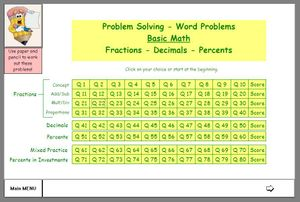You requested it - here it is!
After many requests for dedicated word problem programs designed in the MathMedia style, we are pleased to offer this interactive basic math problem solving program for math students who have mastered the mechanics of the operations on fractions, decimals, percents, pre-algebra, and intro-geometry. With over 200 menu-driven practice problems, students will be able to focus on reading the words, deciphering the meaning, and applying what they know about basic math problem solving. Students will learn math problem-solving strategies and practice the types of math word problems, which typically appear on standardized tests. Hints are available to help and coach the student along. Score reports are generated at the end of each section.
This math problem solving program is divided into topic sections, so that a student who has mastered fractions but not yet pre-algebra can still work on the fraction section before tackling the pre-algebra section. These math word problems are geared toward teaching and learning problem solving techniques on the types of questions encountered on standardized tests given to 5th grade math through 8th grade math students as well as typical everyday life math problems.
There is much talk in Washington about providing our students with 21st-century skills, such as problem solving and critical thinking - this program is part of that learning process for elementary school and middle school students learning to think while using their math skills.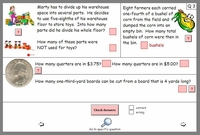Read, think, calculate, and answer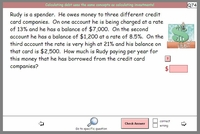Over 300 questions with hints and solutions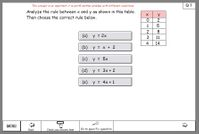Algebra Reasoning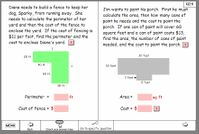Introductory Geometry questions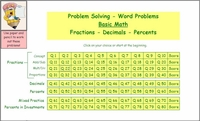Program 6: Word Problems for Basic MathTypical Standardized Test QuestionsRegular price: \$49.00Limited Time Offer: \$24.50 Problem Solving - Topics